Next: Potential due to uniform Up: Newtonian gravity Previous: Gravitational potential energy

# Axially symmetric mass distributions

At this point, it is convenient to adopt standard spherical coordinates,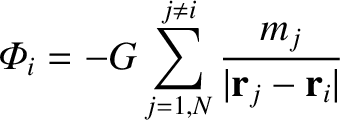, aligned along the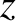-axis. These coordinates are related to regular Cartesian coordinates as follows (see Section A.8):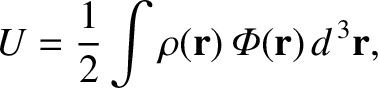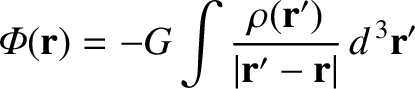(3.24)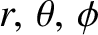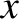(3.25) and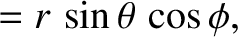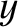(3.26)

Consider an axially symmetric mass distribution; that is, a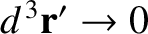that is independent of the azimuthal angle,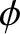. We would expect such a mass distribution to generated an axially symmetric gravitational potential,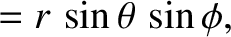. Hence, without loss of generality, we can set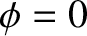when evaluating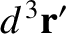from Equation (3.10). In fact, given thatin spherical coordinates, this equation yields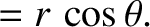(3.27)

with the right-hand side evaluated at. However, because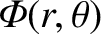is independent of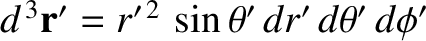, Equation (3.27) can also be written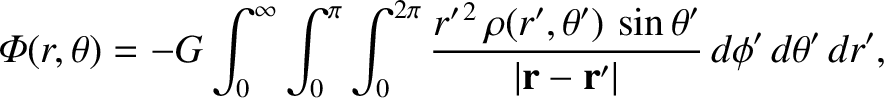(3.28)

where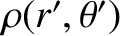denotes an average over the azimuthal angle.

Now,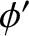(3.29)

and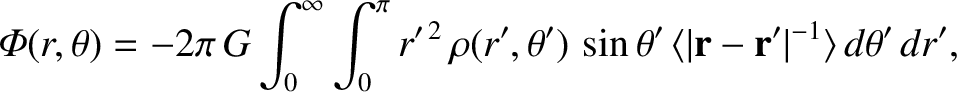(3.30)

where (at)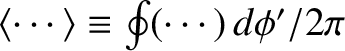(3.31)

Hence,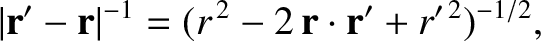(3.32)

Suppose that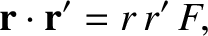. In this case, we can expand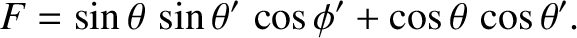as a convergent power series in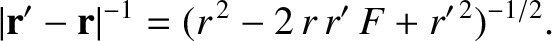, to give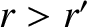(3.33)

Let us now average this expression over the azimuthal angle,. Because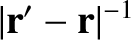,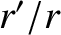, and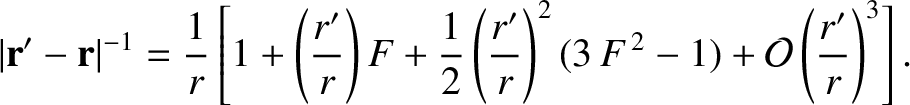, it is easily seen that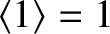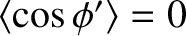(3.34) and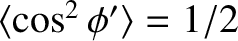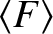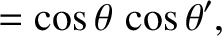(3.35)

Hence,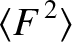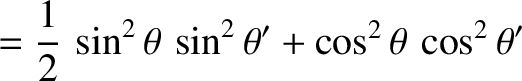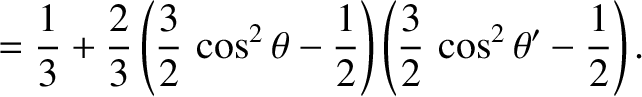(3.36)

Now, the well-known Legendre polynomials,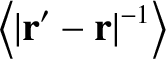, are defined (Abramowitz and Stegun 1965b) as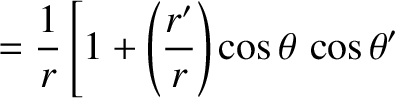(3.37)

for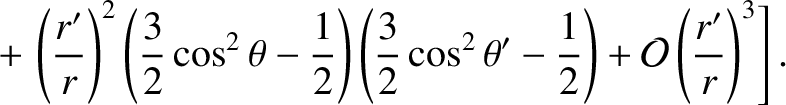. It follows that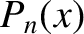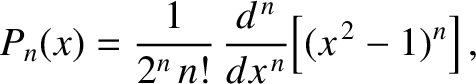(3.38)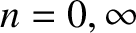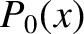(3.39)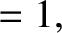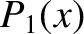(3.40)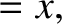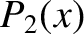(3.41)

and so on. The Legendre polynomials are mutually orthogonal: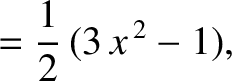(3.42)

(Abramowitz and Stegun 1965b). Here,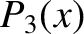is 1 if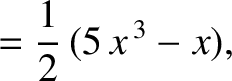, and 0 otherwise. The Legendre polynomials also form a complete set: any function ofthat is well behaved in the interval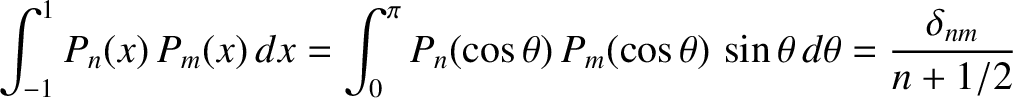can be represented as a weighted sum of the. Likewise, any function of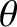that is well behaved in the interval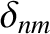can be represented as a weighted sum of the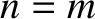.

A comparison of Equation (3.36) and Equations (3.38)-(3.40) makes it reasonably clear that, when, the complete expansion of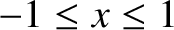is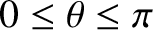(3.43)

Similarly, when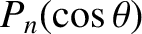, we can expand in powers of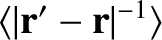to obtain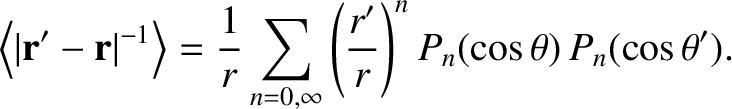(3.44)

It follows from Equations (3.28), (3.43), and (3.44) that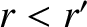(3.45)

where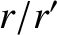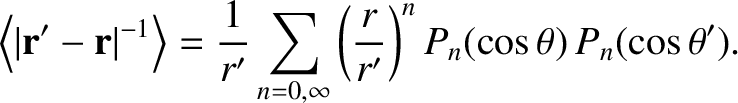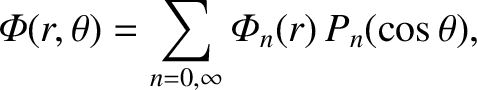(3.46)

Given that theform a complete set, we can always write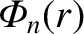(3.47)

This expression can be inverted, with the aid of Equation (3.42), to give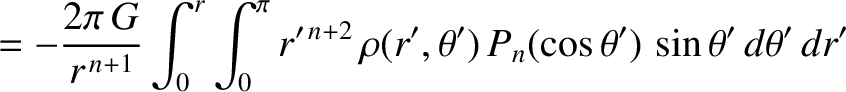(3.48)

Hence, Equation (3.46) reduces to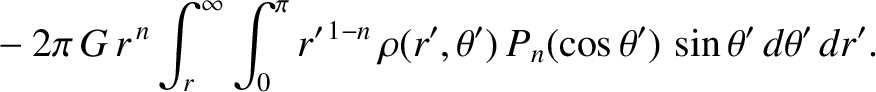(3.49)

Thus, we now have a general expression for the gravitational potential,, generated by an axially symmetric mass distribution,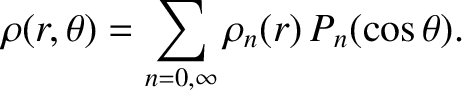.Next: Potential due to uniform Up: Newtonian gravity Previous: Gravitational potential energy
Richard Fitzpatrick 2016-03-31